# CONDITIONAL PROBABILITY

CONDITIONAL PROBABILITY

If A and B are two events, then the conditional probability of event A given that event B has already occured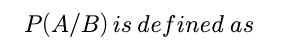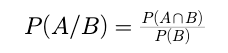Similarly, the probability of B given that A has already occured will be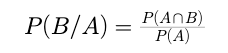Therefore,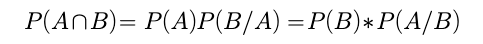Example:

If a pair of dice is thrown and it is known that sum of the numbers is even, then find the probability that the sum is less than 6.

Let A be the given event and let B be the event, whose probability is to be found. Then

Required probability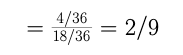Post By : Rahul Kumar 01 Nov, 2020 381 views Maths